#### The two-dimension model. Flaring and escape

In this model by Battaner and Florido (1995) the vertical component of the equation of motion is written as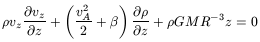(120)

where vz(R, z) is the vertical velocity, vA(R) is the Alfven velocity considered to be a constant in the vertical direction (because of the assumption B2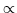, as vA = B/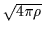); also= p(R, z)/(R, z) is assumed to be constant (isothermal condition, or rather, constant cloud-to-cloud velocity dispersion) and the vertical component of the gravitational force is simplified to that due to a central point mass; this assumption is in part justified because it is assumed that no dark matter halo is present and because we are considering just the outermost part of the disk. Many of these simplifying conditions are not necessary and, indeed, are only justified in a model with exploratory aims.

Therefore the motion in the vertical direction was considered to be the result of four terms: the inertial term, the magnetic, the gravitational and the pressure gradient forces. Horizontal velocities were considered, but the term vR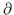vz/z was neglected.

The equation of continuity restricts the possibilities of the vertical flux. It was assumed that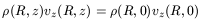(121)

To calculate vz(R, 0) at z = 0 in the plane (or slightly above the plane, to avoid the problem arising from the symmetry in the galactic plane; vz(R,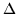z) = - vz(R, -z), for very smallz, this would imply vz(R, 0) = 0) some assumptions must be made; i.e. adopting a physical mechanism responsible for the vertical flux, which could be produced by supernova explosions. In this case it would be preferable to adopt a hypothesis of the type vz(R, 0), if for large time scales supernovae are born where the gas is denser. Battaner and Florido, instead, considered that Parker instabilities were the main origin of the vertical flux and assumed for the flux at z = 0: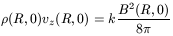(122)

the value of k being considered a free parameter. This condition is equivalent to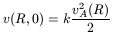(123)

Instead of, it is preferable to use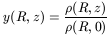(124)

because the calculation of(R, 0) and the profile y(R, z) were made using different equations. Using Alfven's velocity the radial component of the equation of motion is now written as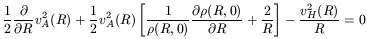(125)

The variable vH(R) is defined by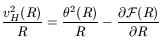(126)

which is what in more conventional theories is called the "halo velocity" as a way of introducing the halo potential. This quantity vH(R) was used because it can be found directly in the literature, but no dark matter halo was introduced. No inertial terms, neither vzvR/z nor vRvR/R, were considered to be important and the gradient pressure force was again considered negligible.

The surface density was adopted from the literature, and therefore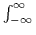(R, z)dz was kept constant. To compensate for the escape, it was assumed that the horizontal flux from the central region was so easily established that it was able to supply the necessary escaped mass at all radii. The function vR(r) was however found to be negligible. When the vertical velocities reached a value higher than the typical velocity dispersion, say 8 km/s, gas clouds were considered to have escaped from the disk, but not necessarily from the galaxy.

Though not affecting the numerical computations, it is interesting to obtain two functions of interest in the interpretation. One of these is the "Flaring Function", Z(R) defined by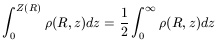(127)

and the other is the total mass loss rate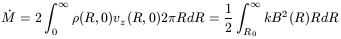(128)

where R0 is the adopted inner boundary radius. Z(R) is important because if the disk is highly magnetized, Z(R) can become unacceptably large. Ifis calculated to be too large, the whole galaxy could evaporate.

A numerical integration of these equations was carried out by Battaner and Florido (1995) taking M31 as a representative galaxy, with a convergent procedure that we do not reproduce here, but just show the results (see Fig. 20).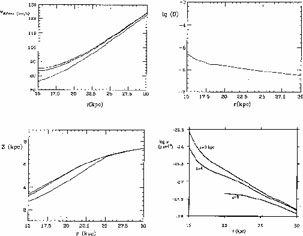Figure 20. Top left: Alfvén's speed in km s-1 for three values of the free parameter k (10-9, upper curve; 3 × 10-9, middle curve; 10-8cm-1s, lower curve). Top right: Magnetic field strength in gauss. Botton left: The flaring function Z(r) in kpc, for three different values of the free parameter k defined in the text (10-9, upper curve; 3 × 10-9, middle curve; 10-8cm-1s, lower curve). Botton right: Density profiles for the value of the free parameter k = 3 × 10-9cm-1s, for three different values of z=0, 4 and 8 kpc from the galactic plane. From Battaner and Florido (1995). Courtesy of the Royal Astronomical Society.

The free parameter k should have a value of between 10-9 and 10-8cm-1s, with k = 3 × 10-9 being the value giving the most reliable results. The mass loss rate,was found to be 0.054, 0.16 and 0.55 M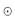yr-1 for k = 10-9, 3 × 10-9 and 10-8cm-1s; this is still rather low, lower in any case than the typical value given by fountain models (15 Myr-1; Kahn, 1994). Part of this gas that escaped from the disk would eventually fall back into the disk. Even if this were not the case and in the worst situation in which all the gas escaping from the disk escaped from the galaxy, the total mass loss during the whole history of the galaxy (assuming the flux to be constant in time) would be of the order of 0.16 Myr-1 × 1010yr1.6 × 109M, an acceptable value.

Even with the simplifying conditions assumed, a coherent general scenario is obtained:

a) The Alfven speed increases outwards is always lower than the rotational velocity but has a common order of magnitude. B anddecrease but B2/increases.

b) The effect of flaring and escape reduces the magnetic field required to drive the rotation of the outer disk. In the previous simple model, 6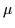G at 30 kpc was obtained. Now it is a full order of magnitude less. This is a very exciting figure, as it confirms that moderate magnetic field strengths can have a decisive influence on the rotation curve.

c) The flaring seems to be high but this is in reasonable agreement with observations. For low radii, the adoption of the central point mass potential is not appropriate. For instance, Z(R) is too high for radii less than about 17 kpc. But in our Galaxy, where precise data exist for very large radii, a value of Z6 kpc at R= 20 kpc from the plots provided by Diplas and Savage (1991) is reproduced by the model fairly well.

d) The densities are reasonable. At 30 kpc, values of the order of 1.6 × 10-28gcm-3 (10-4atoms cm-3) were obtained, and at 25 km, 5 × 10-28gcm-3. In any case these values are compatible with the observed surface density, as this function was adopted from the observations.

e) Velocities (vertical at the base of the galactic plane and radial) are small, of the order of a few km/s, which are nearly undetectable and therefore do not introduce problems of disagreement with any measurement. Consider that 2 km/s at z0 may produce 10 km/s at z8kpc and R20kpc, due to continuity. (The flux would be conserved, so the decrease in density accelerates the vertical speed). Velocities of this order of magnitude are observed even in a quiet disk (see for instance Jiménez-Vicente et al. 1999).